ISEE Lower Level Math : Ratio and Proportion

Example Questions

Example Question #31 : Ratio And Proportion

The scale on a map is 2cm : 12km . If and the actual distance between two lakes is 600 km, find the distance between the lakes as shown on a map.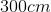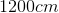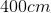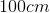Explanation:

To solve this problem, set up the proportion: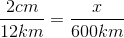Cross multiply: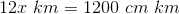Divide both sides by 12 to isolate x.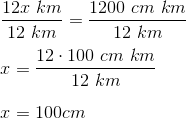Example Question #32 : Ratio And Proportion

You and a friend win a raffle of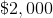.  You and him split it with a ratio of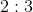since he paid more into the raffle.  What is your share?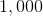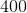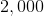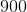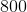Explanation:

Since there are a total of five shares in the ratio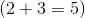and you get two shares, your share isof the total.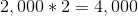and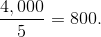Your share is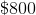.

Example Question #33 : Ratio And Proportion

If three friends start a business and two invested equally while the third invested twice the amount each of them did, what is the ratio for their shares?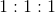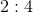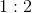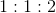Explanation:

Since the first two have to be equal, you can start by representing them byshare apiece.

The third invested twice the amount that each of the others did so you double their share to get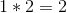.

So the first friend gets one share and so does the second.

The third friend gets two shares and the ratios looks like,.

Example Question #34 : Ratio And Proportion

Sharon hasmarbles,of which are blue andof which are white. Write the ratio of blue marbles to white marbles in simplest form.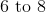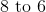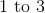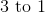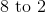Explanation:

The ratio of blue marbles to white marbles is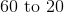, but this is not in simplest form. Rewrite this as a fraction for simplicity, then divide both numbers by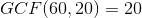: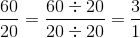In simplest form, the ratio isExample Question #35 : Ratio And Proportion

A fruit punch recipe calls for 1 cup of orange juice, half a cup of pineapple juice, and 2 cups of soda. What is the ratio of orange juice to pineapple juice to soda water?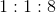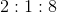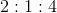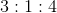Explanation:

If a fruit punch recipe calls for 1 cup or orange juice, half a cup of pineapple juice, and 2 cups of soda, the ratio would be: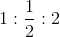Multiply all three numbers by 2: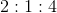Example Question #1 : How To Find A Proportion

A cookie recipe that bakes 12 cookies calls for 3 eggs. How many eggs would be needed to make 36 cookies?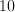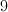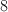Explanation:

36 cookies is 3 times more than 12. Multiply the number of eggs by 3 to get 9.

Example Question #2 : How To Find A Proportion

Give the value ofthat makes this proportion statement correct: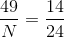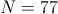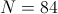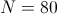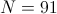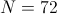Explanation:

Cross-multiply, then solve for: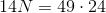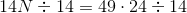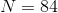Example Question #3 : How To Find A Proportion

Give the value ofthat makes this proportion statement correct: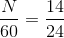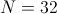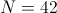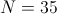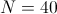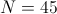Explanation:

Solve forby multiplying both sides by 60: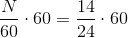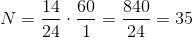Example Question #4 : How To Find A Proportion

If 1 inch on a map represents 5 miles, how far apart, in miles, are two cities that are 6.6 inches apart on the map?

33 miles

12 miles

24 miles

6.6 miles

36 miles

33 miles

Explanation:

Set up a proportion with the inches on the map and physical miles: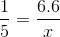Solve for x: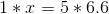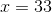Example Question #5 : How To Find A Proportion

A group of 6th grade students at Manor Intermediate are going on a field trip to the local theme park.

There are 2 chaperones required for every 9 students on the field trip.  How many chaperones will need to attend the field trip if there are 27 students going?chaperoneschaperoneschaperones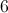chaperoneschaperones

Explanation:

The best way to solve this problem is to set it up as two ratios that are equivalent to each other.  This forms a proportion.  Make sure to set up the ratios so that the relationships go together across the equal sign.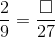Use cross products to then solve for the missing value.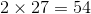Therefore, mental math or division can be used to find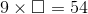.

Since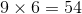,

there would need to be 6 chaperones attending the field trip.